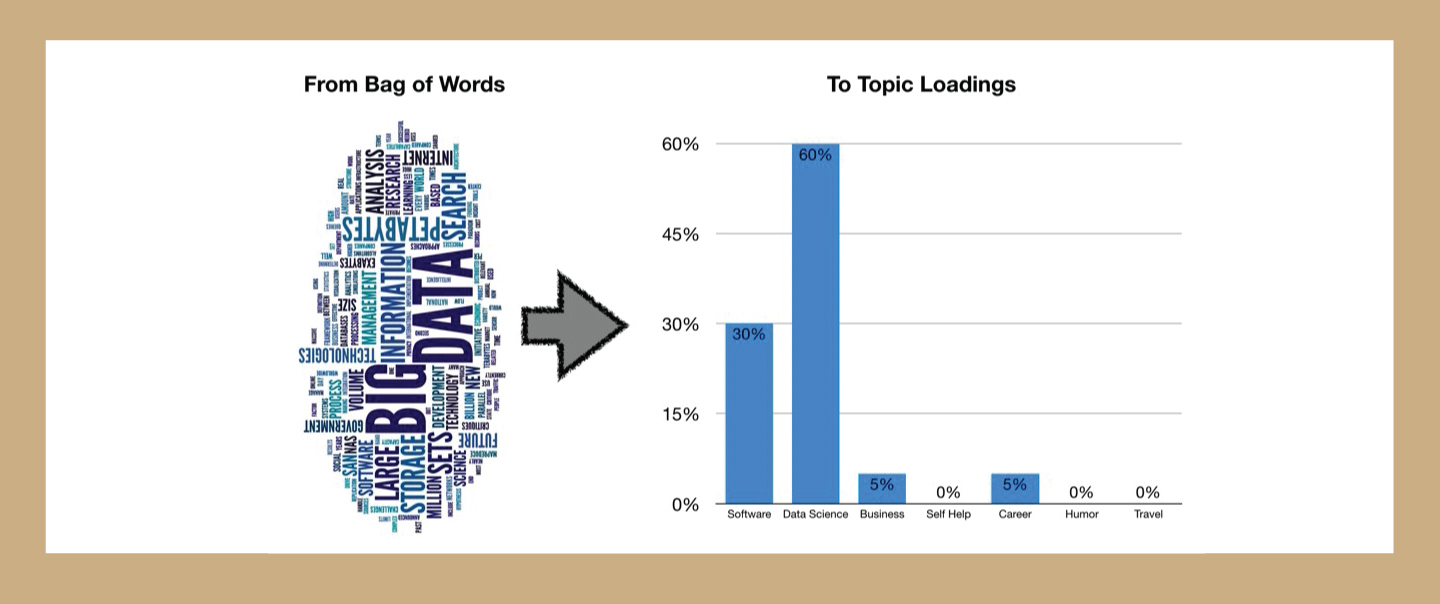## Topic Modeling (2)

In a previous article we have reviewed the example and application of topic modeling. This time we will review the popular approaches of topic modeling.

## Approaches for Topic Modeling

### 1. Latent Semantic Analysis (LSA)

It is based on what is known as the distributional hypothesis which states that the semantics of words can be grasped by looking at the contexts the words appear in. In other words, under this hypothesis, the semantics of two words will be similar if they tend to occur in similar contexts.

That said, LSA computes how frequently words occur in the documents – and the whole corpus – and assumes that similar documents will contain approximately the same distribution of word frequencies for certain words. In this case, syntactic information (e.g. word order) and semantic information (e.g. the multiplicity of meanings of a given word) are ignored and each document is treated as a bag of words.

The standard method for computing word frequencies is what is known as tf-idf. This method computes frequencies by taking into consideration not only how frequent words are in a given document, but also how frequent words are in all the corpus of documents. Words with a higher frequency in the full corpus will be better candidates for document representations than less frequent words, regardless of how many times they appear in individual documents. As a result, tf-idf representations are much better than those that only take into consideration word frequencies at document level.

Once tf-idf frequencies have been computed, we can create a Document-term matrix which shows the tf-idf value for each term in a given document. This matrix will have rows for every document in the corpus and columns for every term considered.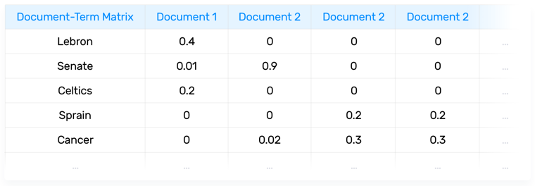This Document-term matrix can be decomposed into the product of 3 matrices (USV) by using singular value decomposition (SVD). The U matrix is known as the Document-topic matrix and the V matrix is known as the Term-topic matrix: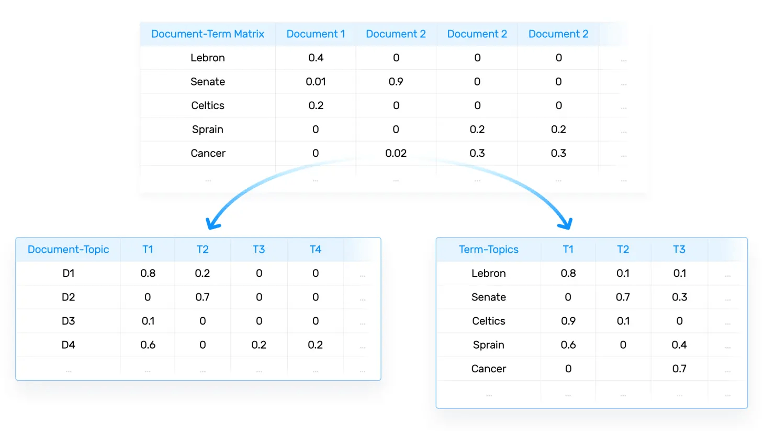Linear algebra guarantees that the S matrix will be diagonal and LSA will consider each singular value, i.e. each of the numbers in the main diagonal of matrix S, as a potential topic found in the documents.

Now, if we keep the largest t singular values together with the first t columns of U and the first t rows of V, we can obtain the t more frequent topics found in our original Document-term matrix. We call this truncated SVD since it does not keep all of the singular values of the original matrix and, in order to use it for LSA, we will have to set the value of t as a hyperparameter.

The quality of the topic assignment for every document and the quality of the terms assigned to each topic can be assessed through different techniques by looking at the vectors that make up the U and V matrices, respectively.

### 2. Latent Dirichlet Allocation (LDA)

Latent Dirichlet Allocation (LDA) and LSA are based on the same underlying assumptions: the distributional hypothesis, (i.e. similar topics make use of similar words) and the statistical mixture hypothesis (i.e. documents talk about several topics) for which a statistical distribution can be determined. The purpose of LDA is mapping each document in our corpus to a set of topics which covers a good deal of the words in the document.

What LDA does in order to map the documents to a list of topics is assign topics to arrangements of words, e.g. n-grams such as best player for a topic related to sports. This stems from the assumption that documents are written with arrangements of words and that those arrangements determine topics. Yet again, just like LSA, LDA also ignores syntactic information and treats documents as bags of words. It also assumes that all words in the document can be assigned a probability of belonging to a topic. That said, the goal of LDA is to determine the mixture of topics that a document contains.

In other words, LDA assumes that topics and documents look like this: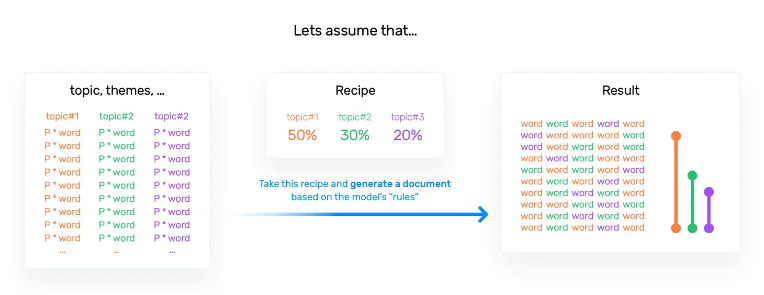And, when LDA models a new document, it works this way: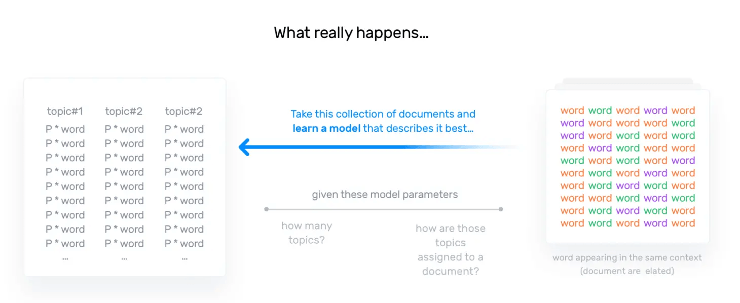The main difference between LSA and LDA is that LDA assumes that the distribution of topics in a document and the distribution of words in topics are Dirichlet distributions. LSA does not assume any distribution and therefore, leads to more opaque vector representations of topics and documents.

There are two hyperparameters that control document and topic similarity, known as alpha and beta, respectively. A low value of alpha will assign fewer topics to each document whereas a high value of alpha will have the opposite effect. A low value of beta will use fewer words to model a topic whereas a high value will use more words, thus making topics more similar between them.

A third hyperparameter must be set when implementing LDA, namely, the number of topics the algorithm will detect since LDA cannot decide on the number of topics by itself.

The output of the algorithm is a vector that contains the coverage of every topic for the document being modeled. It will look something like this [0.2, 0.5, etc.] where the first value shows the coverage of the first topic, and so on. If compared appropriately, these vectors can give you insights into the topical characteristics of your corpus.

### 3. Topic Modeling with BERT

Bidirectional Encoder Representations from Transformers is a technique for natural language processing pre-training developed by Google. Steps involved for topic modeling using BERT are described below.

##### a.Embeddings

The very first step we have to do is converting the documents to numerical data. We use BERT for this purpose as it extracts different embeddings based on the context of the word. Not only that, but there are also many pre-trained models available ready to be used.

##### b. Clustering

We want to make sure that documents with similar topics are clustered together such that we can find the topics within these clusters. HDBSCAN is a density-based clustering algorithm that works quite well with UMAP since UMAP maintains a lot of local structure even in lower-dimensional space. Moreover, HDBSCAN does not force data points to clusters as it considers them outliers.

NOTE: You could skip the dimensionality reduction step if you use a clustering algorithm that can handle high dimensionality like a cosine-based k-Means.

##### c. Topic Creation

To derive topics from clustered documents, we can use a class-based variant of TF-IDF (c-TF-IDF), that would allow to extract what makes each set of documents unique compared to the other.

Apply the class-based TF-IDF:Class-based TF-IDF by joining documents within a class, where the frequency of each word t is extracted for each class i and divided by the total number of words w. This action can be seen as a form of regularization of frequent words in the class. Next, the total, unjoined, number of documents m is divided by the total frequency of word t across all classes n

Now, we have a single importance value for each word in a cluster which can be used to create the topic. If we take the top 10 most important words in each cluster, then we would get a good representation of a cluster, and thereby a topic.

Note: Class is unsupervised clusters

In a previous article and this article, we have reviewed the notation, use case, application and popular approaches. We will review the usage of the popular libraries for topic modeling in our next article.